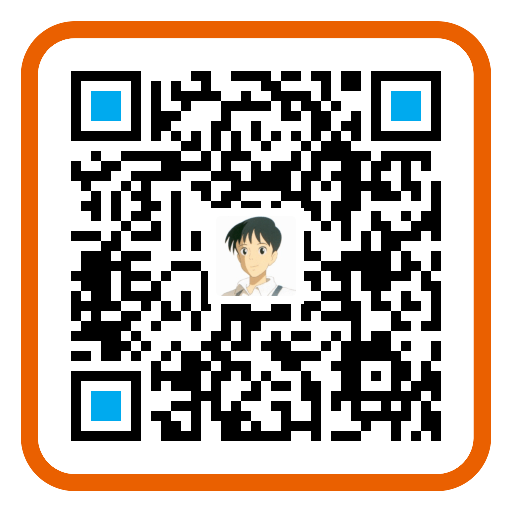SuperPoint: Self-Supervised Interest Point Detection and Description

#### Motivation

Self-Supervised 是以 From Simple to Complex 的方式实现的，之所以这么做，在于作者相信能够 transfer knowledge from a synthetic dataset onto real-world images，具体步骤如下图所示：

1. 首先是 pre-train an initial interest point detector on synthetic data（这是 From Simple to Complex 的 Simple 阶段），这个阶段得到的 Detector 叫作 MagicPoint；MagicPoint 在 synthetic data 上表现比传统的特征点检测子要来得好，但对于 real images，相比于经典检测子还是会 misses many potential interest point locations. 后面这个能力会通过 Homographic Adaptation 这个 multi-scale, multi-transform technique 来补全（意思就是说作者认为，synthetic data 相比于 real images，缺少 multi-scale, multi-transform，所以 MagicPoint 缺的是在 multi-scale, multi-transform 下的鲁棒性？）
2. 第二步是 Interest Point Self-Labeling，现在 unlabeled image（这是 real images，不是 synthetic data，这是 From Simple to Complex 的 Complex 阶段）上检测出特征点，然后再运用 Homographic Adaptation，就可以得到单应性变换后的特征点。Homographic Adaptation 的核心在于 刻画 repeatability，最后 detectors and descriptors 的 loss 其实惩罚的就是在 repeatability 上表现不好的，而非检测的特征点与 Human Annotated 特征点之间的 Loss。也就是说，之所以能够 Self-Supervised，在于 Loss 由刻画 Prediction 与 Groundtruth 之间的差距，变成了刻画 Prediction 在 Homographic Adaptation 下的 repeatability，也就是 Homographic Adaptation 前后的差距。
3. 最后就是 Joint Training，根据 Loss Function 用 ADAM 优化即可，在优化 Detector 的同时 Description 也学了，Description 就是 CNN 最后的特征表示。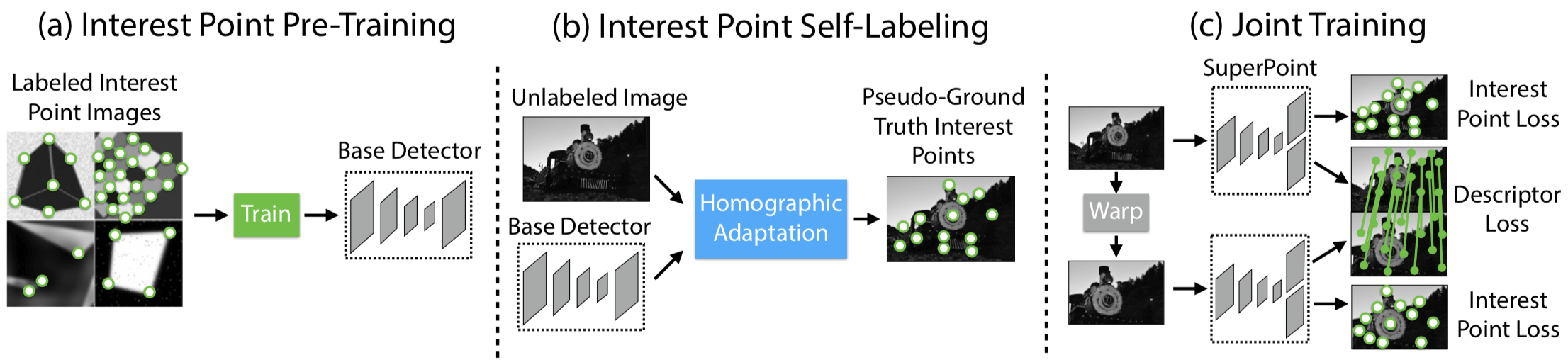#### Model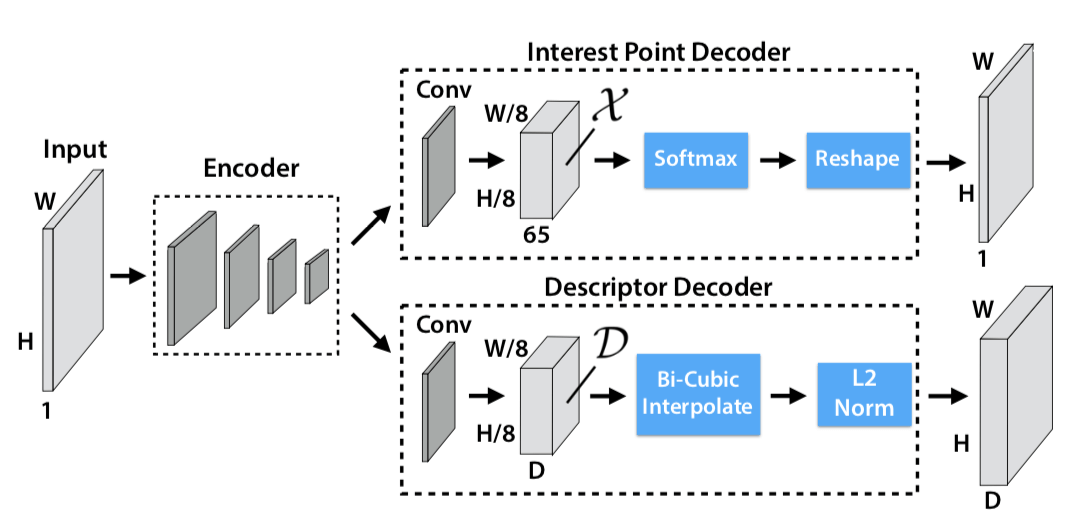upsampling 没有学习，直接用的插值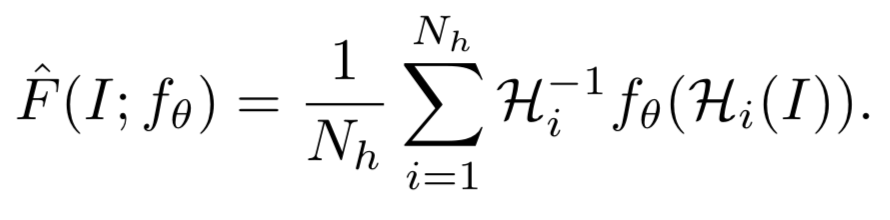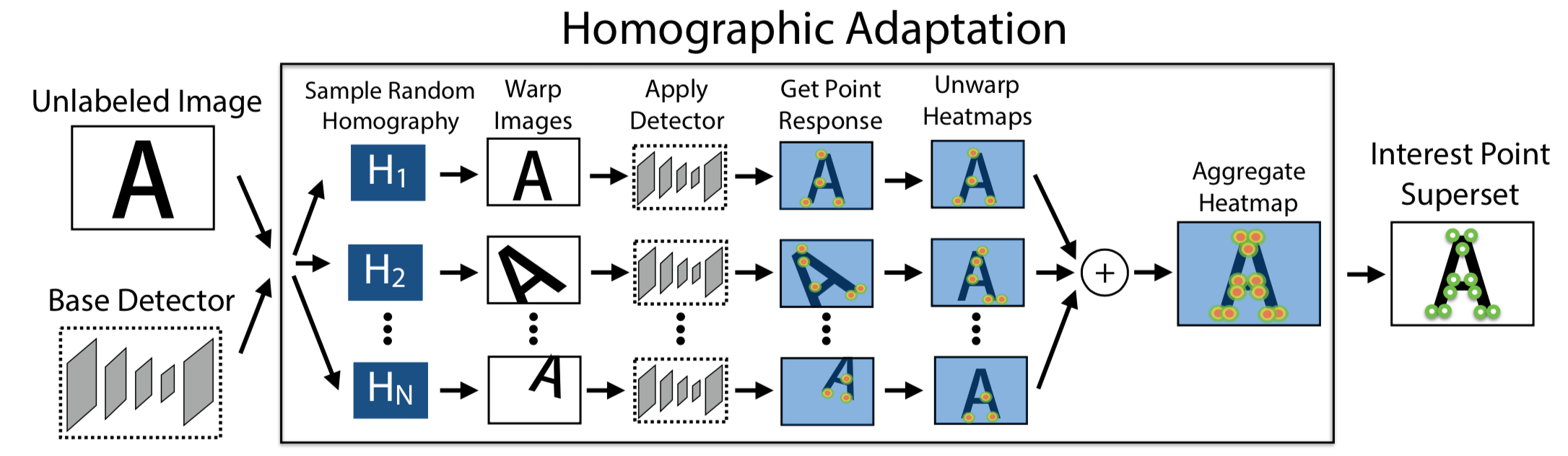#### Loss

$$\begin{array} { l } { \mathcal { L } \left( \mathcal { X } , \mathcal { X } ^ { \prime } , \mathcal { D } , \mathcal { D } ^ { \prime } ; Y , Y ^ { \prime } , S \right) = } \ { \mathcal { L } { p } ( \mathcal { X } , Y ) + \mathcal { L } { p } \left( \mathcal { X } ^ { \prime } , Y ^ { \prime } \right) + \lambda \mathcal { L } _ { d } \left( \mathcal { D } , \mathcal { D } ^ { \prime } , S \right) } \end{array}$$

#### interest point detector loss

interest point detector loss 具体如下

$$\mathcal { L } { p } ( \mathcal { X } , Y ) = \frac { 1 } { H { c } W { c } } \sum { h = 1 \atop w = 1 } ^ { H { c } , W { c } } l { p } \left( \mathbf { x } { h w } ; y _ { h w } \right)$$

$$l { p } \left( \mathbf{ x } { h w } ; y \right) = - \log \left( \frac { \exp \left( \mathbf { x } { h w y } \right) } { \sum { k = 1 } ^ { 65 } \exp \left( \mathbf { x } _ { h w k } \right) } \right)$$

$y { h w }$ 是 label，是特征点在这个 $8 \times 8$ 的 Cell 里面的哪个 pixel 的 label；$\mathbf { X } { h w }$ 是一个长度为 65 的特征向量，里面的每一个数值代表着相应的 pixel 上是特征点的响应值（只是响应值不是概率，经过上式 的 softmax 后才是概率值）；上式是典型的 Cross Entropy Loss，因此 interest point detector loss 其实是一个 Cross Entropy Loss。

Y 就是 MagicPoint 在原图上检测出的兴趣点，作为 pseudo groundtruth interest point，X 则是 SuperPoint 网络的 Detector 在原图上检测出的兴趣点，在第一次迭代的时候，X 和 Y 应该是一样的，因为 MagicPoint 就是没有 descriptor head 的 SuperPoint，但随着 SuperPoint 的迭代优化，X 是会发生变化的。这个时候这个 Loss 会去惩罚跟 Y 不一样的 X，也许 X 检出了更多的点呢，这一点合理吗？

MagicPoint performs reasonably well on real world images 但还不够好，相比于其他经典检测子，但是 Homographic Adaptation 也就是 self-supervised approach for training on real-world images 提高了性能（提高性能不一定非要从减小 Prediction 和 Groundtruth Annotation 的任务中来，也可以是构建其他 Loss，这就是 Self-Supervised 的精髓吧 ）

#### descriptor loss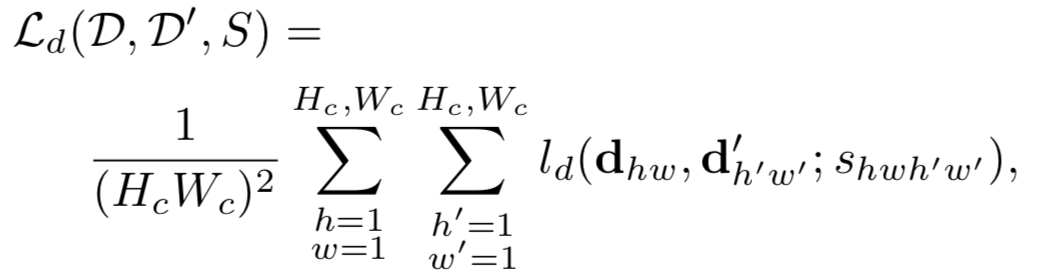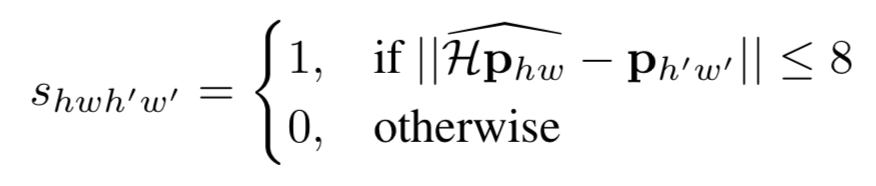$$\mathcal{ H } \mathbf { x } = f _ { \theta } ( \mathcal { H } ( I ) )$$

\begin{aligned} l { d } \left( \mathbf { d } , \mathbf { d } ^ { \prime } ; s \right) & = \lambda { d } s \max \left( 0 , m { p } - \mathbf { d } ^ { T } \mathbf { d } ^ { \prime } \right) \ & + ( 1 - s ) * \max \left( 0 , \mathbf { d } ^ { T } \mathbf { d } ^ { \prime } - m { n } \right) \end{aligned}